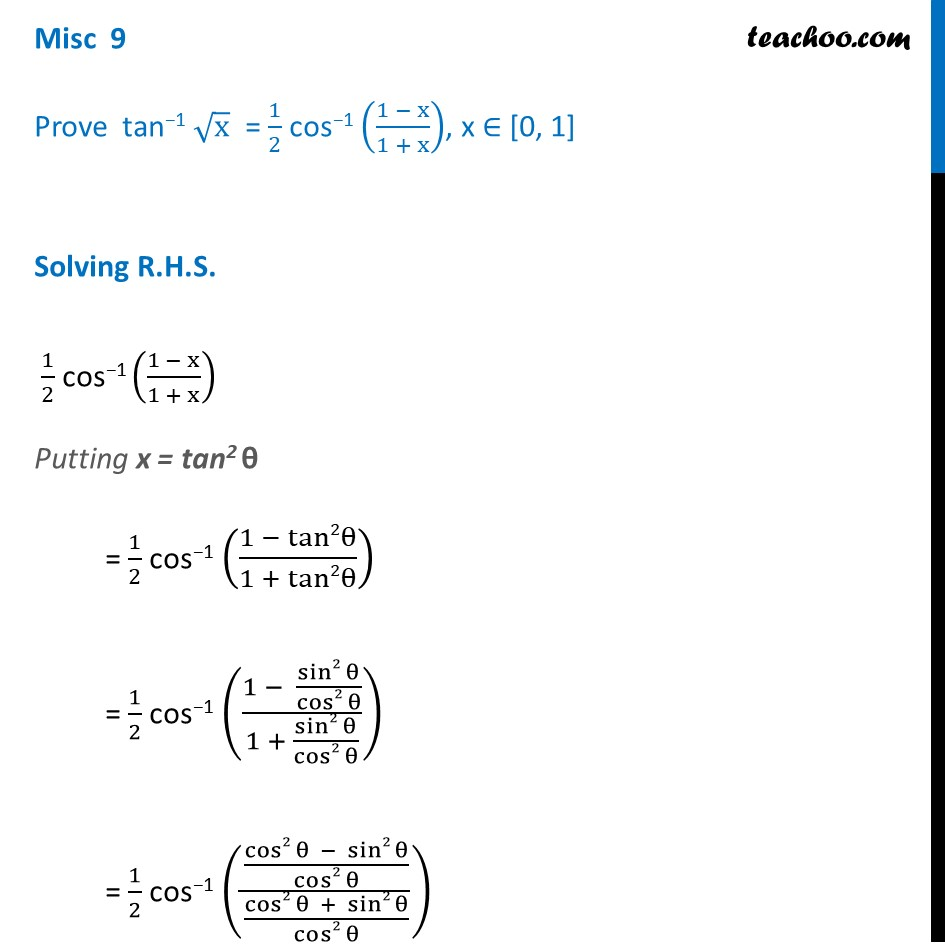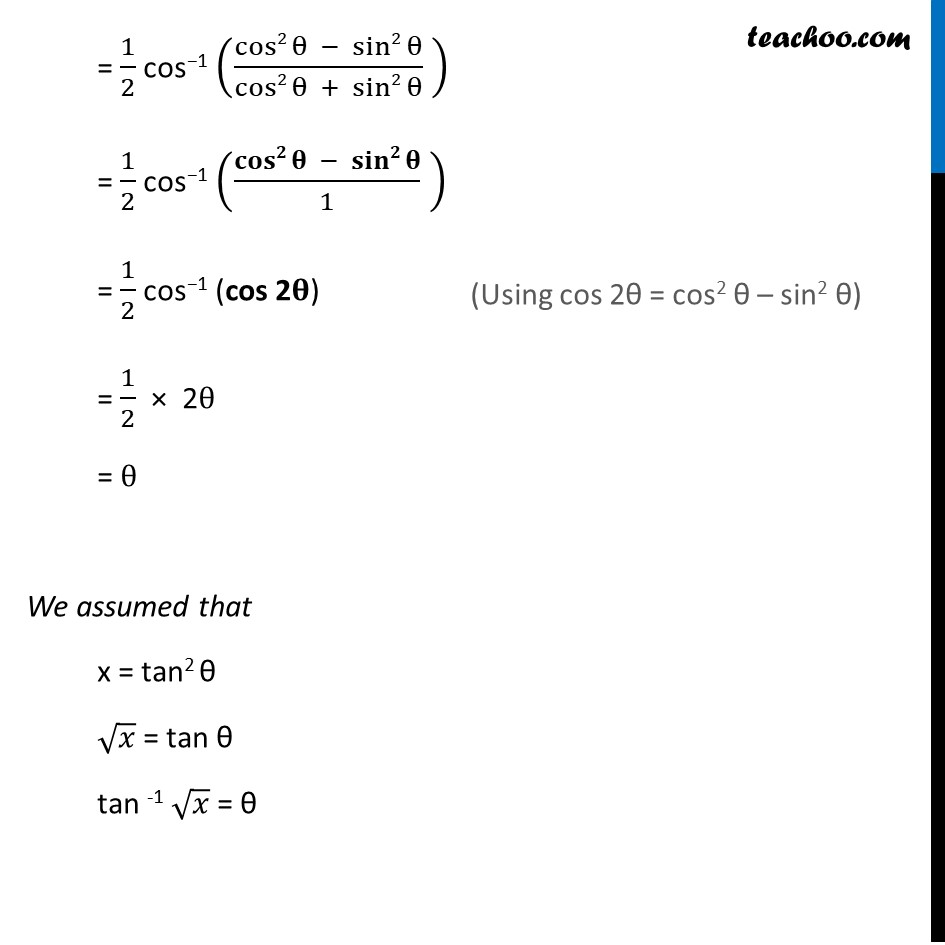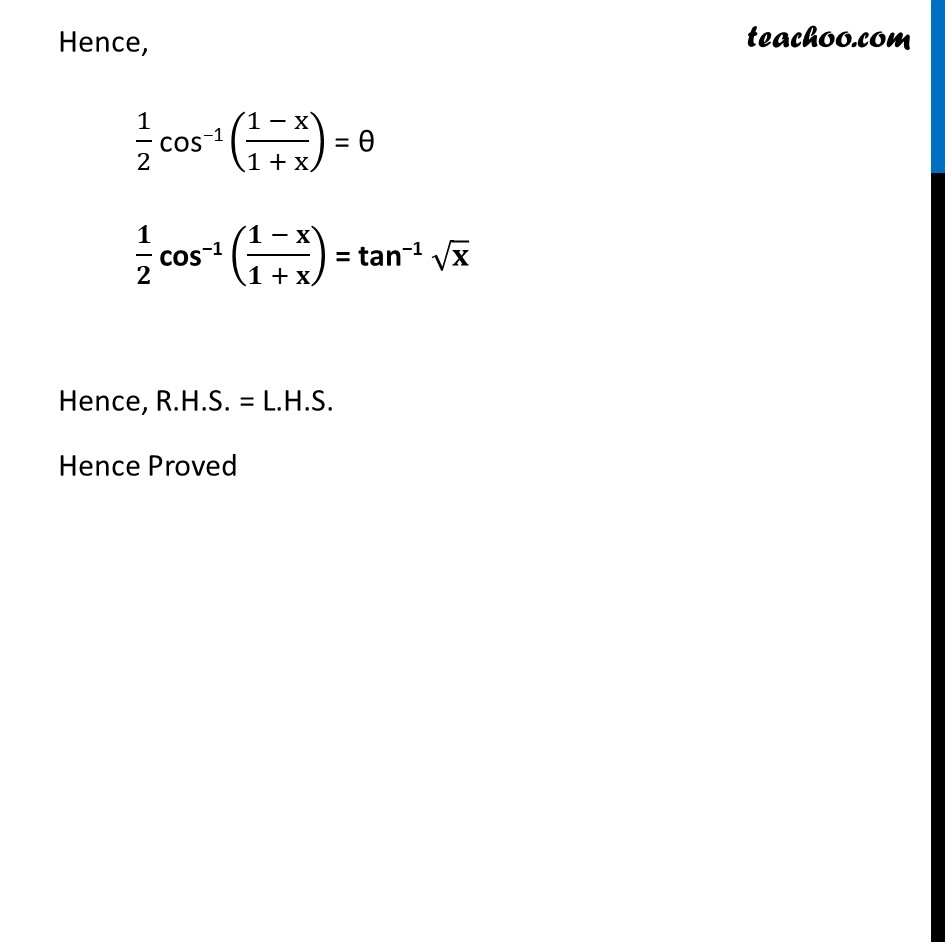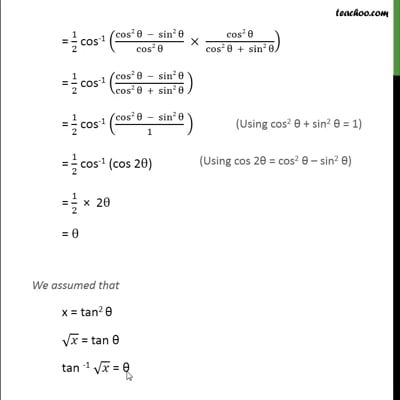Miscellaneous

Chapter 2 Class 12 Inverse Trigonometric Functions
Serial order wiseThis video is only available for Teachoo black users

Solve all your doubts with Teachoo Black (new monthly pack available now!)

### Transcript

Misc 9 Prove tan−1 √x = 1/2 cos−1 ((1 − x)/(1 + x)), x ∈ [0, 1] Solving R.H.S. 1/2 cos−1 ((1 − x)/(1 + x)) Putting x = tan2 θ = 1/2 cos−1 ((1 − tan2θ)/(1 + tan2θ)) = 1/2 cos−1 ((1 − (sin2 θ)/(cos2 θ))/(1 + (sin2 θ)/(cos2 θ))) = 1/2 cos−1 (((cos2 θ − sin2 θ)/(cos2 θ))/((cos2 θ + sin2 θ)/(cos2 θ))) = 1/2 cos−1 ((cos2 θ − sin2 θ)/(cos2 θ + sin2 θ) ) = 1/2 cos−1 ((𝐜𝐨𝐬𝟐 𝛉 − 𝐬𝐢𝐧𝟐 𝛉)/1 ) = 1/2 cos−1 (cos 2𝛉) = 1/2 × 2θ = θ We assumed that x = tan2 θ √𝑥 = tan θ tan -1 √𝑥 = θ (Using cos 2θ = cos2 θ – sin2 θ) Hence, 1/2 cos−1 ((1 − x)/(1 + x)) = θ 𝟏/𝟐 cos−1 ((𝟏 − 𝐱)/(𝟏 + 𝐱)) = tan−1 √𝐱 Hence, R.H.S. = L.H.S. Hence Proved